Journal of Electrical and Electronic Engineering
Volume 4, Issue 5, October 2016, Pages: 114-119

Propagation of Photon in Multilayer Anisotropic Metamaterials

Yunxia Dong

School of Electrical and Electronic Engineering, North China Electric Power University, Beijing, China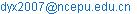Yunxia Dong. Propagation of Photon in Multilayer Anisotropic Metamaterials. Journal of Electrical and Electronic Engineering. Vol. 4, No. 5, 2016, pp. 114-119. doi: 10.11648/j.jeee.20160405.15

Received: October 11, 2016; Accepted: November 5, 2016; Published: November 10, 2016

Abstract: We present a theoretical study of the propagation properties of polarized photons transmitting through a multilayer cavity with anisotropic metamaterials. We find that there are the resonant peaks of transmission appearing for photons polarized in a certain direction transmitting through the cavity with the anisotropic metamaterials having negative elements of the permittivity tensor. The resonant peak of transmission for photons can be achieved by adjusting the thicknesses of the cavity. The frequency of the resonant peak moves to the lower frequency as the thickness of the cavity which is the air in our designed structure increasing for a three layer cavity. The result also shows that we can adjust the resonant peak through changing the layer number. Increasing the layer number the more resonant peaks appear. The three layer system has one resonant peak. The five layer system has two resonant peaks and the seven layer system has three resonant peaks. The cavity number which also is the number of the air layer decides the resonant peaks. Increasing the cavity thickness the space of the resonant peaks will decrease. For a three layer cavity, the resonant peak will appear to a certain cavity thickness when we keep the frequency of the input photon as a constant. As the frequency of the input photon becomes bigger the thickness of the cavity corresponding to the resonant peak becomes smaller. At the same time the resonant peak becomes sharp. The result shows that the transmission is almost invariant with the changing of the thickness of the anisotropic metamaterials. This means the resonant peak is insensitive to the thickness of the anisotropic metamaterials. So the thickness of the cavity (air) is important to the design of the cavity structure. These conclusions will give us some instructions to the design of the cavity structure with the anisotropic metamaterials. The cavity structures with the anisotropic metamaterials have the potential for applying such as filters for photons with certain polarizations.

Keywords: Anisotropic Metamaterial, Multilayer, Cavity, Resonant Transmission, Polarized Photon

Contents

1. Introduction

Recently, the metamaterials have attracted a great deal of attention from both theoretical and experimental sides. The metamaterials have the artificial structure and the electromagnetic parameters are dependent on the resonant of the electric and the magnetic field. In some frequency region the effective permittivity and the permeability of the materials can be simultaneously negative and the materials are also called as the left-handed materials because the electric field, the magnetic field and the wave vector form a left-handed relation in the materials. Metamaterials composed of periodically positioned scattering elements exhibit a number of peculiar electromagnetic properties, including, for instance, negative refractive indices , amplification of evanescent waves , subwavelength cavity resonators , and zero-averaged refractive-index band gaps  that do not appear in common materials. It was shown experimentally that a metamaterial composed of periodically positioned scattering elements; all conductors could be interpreted as having simultaneously negative permittivity and permeability. A media with isotropic simultaneously negative permittivity and permeability supports propagating solutions whose phase and group velocities are antiparallel. Thus such a metamaterial can be rigorously described as having a negative index of refraction. An experiment about the observation of the negative refraction was reported using a metamaterial composed of wires and split ring resonators deposited lithographically on circuit board material.

Because the unit resonance structures of metamaterials usually are anisotropic , researchers have become interested in their anisotropic properties and have revealed many intriguing phenomena in different kinds of anisotropic metamaterials . Compared with the isotropic materials, anisotropic materials have more freedom to control the propagation and polarization of the electromagnetic waves. People have shown that the property of negative refraction is not confined to materials with negative definite permittivity tensor and permeability tensor, but can be expected to occure in certain classes of uniaxially anisotropic media. Research has shown that electromagnetic wave polarizations can be manipulated freely through reflections by anisotropic metamaterials [10, 11]. A single metamaterial plate can serve as a bandpass filter, transparent wall, and polarization converter under illumination from differently polarized waves . Related research also focuses on the classical electromagnetic wave properties of anisotropic metamaterials. Quantization is required to deal with nonclassical radiation, such as the entangled photon pairs. Based on the quantization scheme of the radiation fields in metamaterials, the quantum optical correlation through an isotropic metamaterial plate has been studied . A study of the quantization of the electromagnetic field in anisotropic metamaterials has also been performed; the input-output relations of quantized radiation have been derived for a single anisotropic metamaterial plate [14, 15]. Some unusual properties for the polarized photon transmitting through a three layer cavity containing anisotropic metamaterials are studies in Ref. . But the research about the polarized photon propagating through a multilayer cavity is not studied at present. It is meaningful to study the propagation of polarized photons through multilayer structures, such as the multilayer cavity of anisotropic metamaterials. In this work, we are interested in the optical anisotropy and the transmission properties at a planar multilayer cavity with a negative permittivity, whose wave characteristics have yet to be examined.

In this work, we study the propagation of polarized photons through the cavity structure with anisotropic metamaterials. Our calculations are based on the phenomenological quantization theory. First we give the theory for the polarized photon transmitting through a multilayer system with the anisotropic metamaterials briefly. Then we give some concrete calculations for different cavity structure. The effect of the layer number and the thickness of the cavity to the transmission resonant peak are considered. Some interesting conclusions are obtained for the multilayer cavity with the anisotropic metamaterials. The rest of this paper is arranged as follows. Section 2 briefly provides the theory of photon propagation through a multilayer structure composed of different anisotropic metamaterials. The numerical results and discussion appear in Sec. 3. Finally, Sec. 4 summarizes the main points of the paper.

2. Theory

We consider a cavity structure composed of different anisotropic metamaterials and isotropic materials. The cavity structure which is placed between [z1, z2] is composed of anisotropic metamaterial plates and the air, as shown in Fig. 1. The air is located between two metamaterial layers, so the air layer can be called as the cavity. Thus we can call the three layer system has one cavity and the five layer system has two cavities. To simplify the proceeding analysis, we assume that the permittivity tensor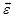and permeability tensor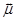of the anisotropic media are given by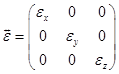and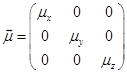.

Such a metamaterial can be readily constructed with elements of either algebraic sign . In fact, the scattering elements comprising the metamaterials used to demonstrate negative refraction are building blocks for the classes of materials to be discussed here. For absorbing anisotropic media, εj and μj ( j = x, y, z) are complex numbers. In order to study the transmission of the polarized photon through such a system, we first need to obtain input-output relations for polarized photon transmitting through such a system. The input-output relations of polarized photon through a single-layer structure with the anisotropic metamaterials have been given in Ref. [15, 16]. Generalizing these input-output relations to a multilayer system is straightforward. Here we provide the main derived process for the calculation of the transmission of the polarized photon propagating through the multilayer structure with the anisotropic metamaterials.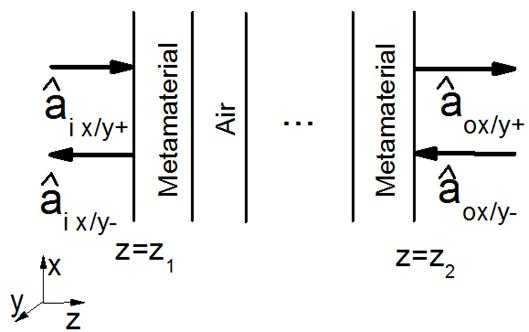Figure 1. The illustration of the cavity structure with the anisotropic metamaterials and the amplitude operators of polarized photon.

The operator-valued Maxwell equations for anisotropic medium free of external sources in frequency space are given as follows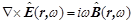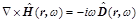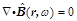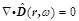where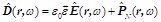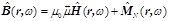Substituting Eqs. , and into Eq. , we get the following equation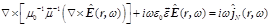Introducing the vector potential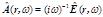, we getThe solution of Eq. can be given by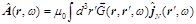where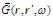is the Green tensor satisfying the equation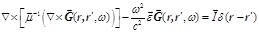Here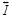is the unit tensor.

Let us first focus on photons linearly polarized in the x direction and y direction and propagating in the z direction. The amplitude operatorsrepresent the incoming photons (+) and outgoing photons (−) polarized in the x/y-direction from the left side of the anisotropic metamaterial. The amplitude operators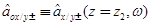correspond to the incoming photons (−) and outgoing photons (+) from the right side, as shown in Fig. 1. Here the subscripts "+" and "−" represent the photons propagating to the right and the left, respectively. The relations between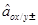and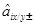are determined by the definition of the vector potential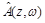and the boundary continuity conditions at the interface of the multilayer structure. Based on the work in Ref. , the input-output relations for the amplitude operators through the multilayer structure can be obtained from.

The matrix T (ω) is block-diagonalizable because both the permittivity and permeability tensors are diagonalizable. If the photon is incident normally from the left side, the transmissions for the photons linearly polarized along the x-direction and y-direction are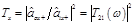and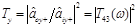, respectively. If the parameters of the cavity are known, the calculation of the transmission of the different polarized photon through such a structure is straightforward.

3. Numerical Results and Discussion

In this section, we present numerical results for the polarized photon through the cavity structure, as shown in Fig. 1. The elements of permittivity and permeability of the tensors of the anisotropic metamaterial are given by εx = 3+i10-6, εy = -3+i10-6, and εz =μx = μy =μz =1. We introduce a small imaginary part to describe the absorption of the metamaterial, with the symbol ‘i’ representing the imaginary unit. Given the rapid development of nanofabrication techniques, the anisotropic metamaterials having permittivity or permeability tensors with elements of either algebraic sign can be achieved by using a periodic array of H-shaped metallic patterns printed on a circuit-board slab . The parameters of the air are given by εx = εy = εz = 1 andμx=μy=μz= 1.

The numerical results for the transmission, as a function of frequency, of photons polarized in the x-direction and y-direction through a single layer anisotropic metamaterials and a three layer cavity structure with the anisotropic metamaterials are shown in Fig. 2 (a) and (b) respectively. The thickness of the single layer anisotropic metamaterial plate is taken to be lMeta = 0.002 m. From the result we find that the transmission Tx is fluctuant with the change of the frequency and the transmission Ty is decreasing with the increasing of the frequency. This is because the component of the permittivity tensor in the x direction is positive and either for the y direction. This means negative permittivity results the absorption. For the three layer cavity, the thickness of each anisotropic metamaterial plate and the thickness of the air are taken to be lMeta = 0.002 m and lair = 0.002 m, respectively. The trend of the transmission Ty is also decreasing with the increasing of the frequency. But a sharp peak appears for the transmission Ty for the frequency about 50 GHz. The transmission Tx is fluctuant with the change of the frequency and does not show any resonant peaks which is similar as the single layer system. The reason is the different sign of the element of the permittivity. This means that we can use the negative permittivity to realize the resonant transmission. The result indicates the potential for applying such cavity structures as filters for photons with certain polarizations.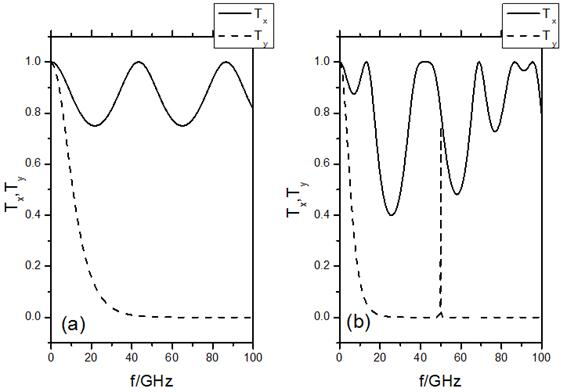Figure 2. The transmissions Tx (solid line) and Ty (dashed line) each as a function of frequency for a single layer metamaterials (a) with lmeta = 0.002 m, and a three layer cavity structure (b) with metamaterial (lmeta = 0.002 m, lair = 0.002 m).

The numerical results for the transmission, as a function of frequency, of photons polarized in the y-direction through a three layer cavity structure with the anisotropic metamaterials are shown in Fig. 3 for different cavity thickness. The thickness of the middle layer which is just the air and can be called as the cavity is taken to be lair = 0.002 m (solid line) and lair = 0.0025 m (dashed line). The thickness of the anisotropic metamaterials is taken to be lMeta = 0.002 m for two different cases. From the result we find the frequency of the resonant peak move to the lower frequency as the thickness of the air increasing. This means we can adjust the resonant frequency of the input photon through changing the thickness of the air.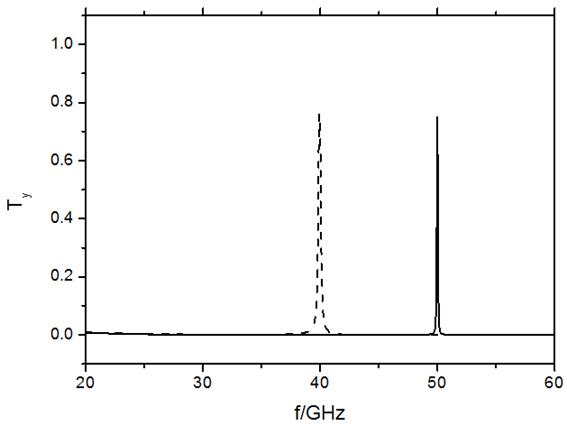Figure 3. The transmissions Ty as a function of frequency for a three layer cavity structure with lmeta = 0.002 m and lair = 0.002 m as solid line and lmeta = 0.002 m and lair = 0.0025 m as dashed line.

We also study the relation between the resonant transmission and the layer numbers. For example, the transmission of the cavity structure with three, five and seven layers is plotted in Fig. 4. The thickness of each metamaterial plate and the thickness of the air are taken to be lMeta = 0.002 m and lair = 0.002 m, respectively. The Fig. 4 (a) shows the result for three layer cavity and the Fig. 4 (b) shows the result for the five layer cavity. The Fig. 5 (c) shows the result for the seven layer cavity. The result is interesting. The three layer system has one resonant peak. The five layer system has two resonant peaks and the seven layer system has three resonant peaks. This means that the cavity number decides the resonant peaks. The three layer system has one cavity. The five layer system has two cavities and the seven layer system has three cavities. Here the cavity is the air. The frequency of the resonant peak of the three layer system is about 50 GHz. The frequencies of the two resonant peaks of the five layer system are located at the two sides of 50 GHz and the values of the two resonant frequencies are about 49.4 GHz and 50.6 GHz. The frequencies of the three resonant peaks of the seven layer system are about 49.2 GHz, 50 GHz and 50.7 GHz. If we add the layer number, more resonant peaks appear. This means that we can adjust the numbers of the resonant peaks through changing the layer number. Increasing the layer number more resonant peaks appear.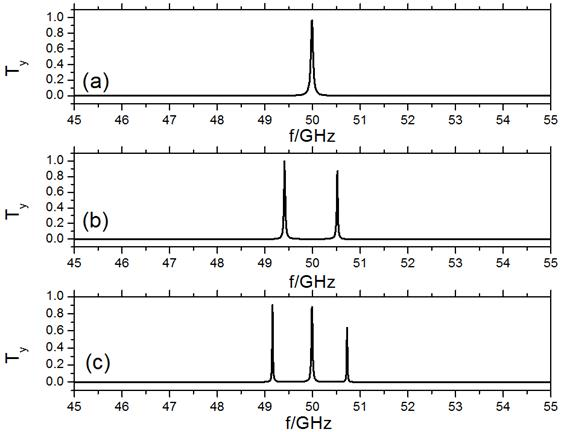Figure 4. The transmissions Ty as a function of frequency for a three layer cavity with anisotropic metamaterials (a), five layer (b) and seven layer (c).

Then we study the relation between the space of the resonant peaks and the cavity thickness. For example, we plot the transmission of the cavity structure with five layers structure in Fig. 5 for several different cavity thicknesses. We call the middle layer which is the air in our designed system as the cavity. The Fig. 5 (a) is corresponding to the structure with lcavity as 0.0025 m and the Fig. 5 (b) is corresponding to the structure with lcavity as 0.002 m. The Fig. 5 (c) is corresponding to the structure with lcavity as 0.0015 m. The thickness of the other four layers is taken to be 0.002 m for three different cases in our calculation. From the plots we find that the space of the resonant peaks will decrease with the increase the cavity thickness. So the thickness of the cavity decides the space of the resonant peaks. If we want to change the location of the resonant peak of the input photon, we can adjust the thickness of the cavity. This conclusion will give instructions to the design of the cavity structure with the metamaterials.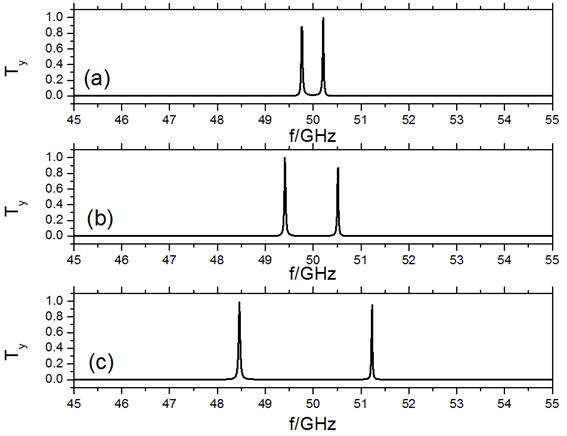Figure 5. The transmissions Ty as a function of frequency for a five layer structure with metamaterials with lcavity = 0.0025 m (a), lcavity = 0.002 m (b) and lcavity = 0.0015 m (c).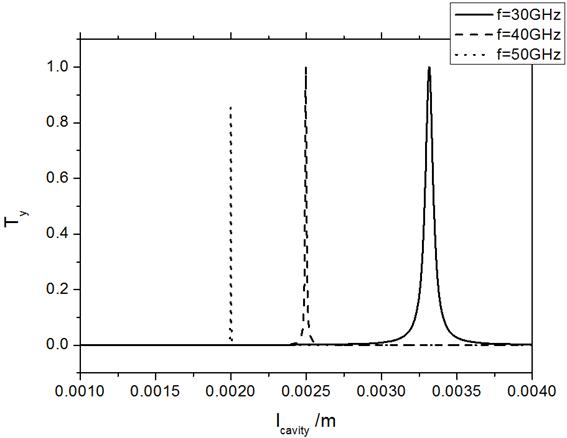Figure 6. The transmissions Ty as a function of lcavity for a three layer structure with metamaterials with frequency f = 30 GHz (solid line), f = 40 GHz (dashed line) and f = 50 GHz (dotted line). The thickness of the metamaterial is taken to be lmeta = 0.002 m for three different frequencies.

Now we study the relations between the resonant peaks and the thickness of the cavity for a three layer cavity. The middle layer which is called as the cavity is the air. The results for the transmissions as a function of the thickness of the cavity which can be written as lcavity for the photon polarized in the y direction with a given frequency are shown in Fig. 6. The results for the frequency of the input photon as f = 30 GHz, f = 40 GHz and f = 50 GHz are shown as the solid line, dashed line and dotted line respectively. The thickness of the metamaterial is taken to be lmeta = 0.002 m for three different frequencies in our calculation. There is the resonant peak appearing in any cases. This means that if the frequency of the input photon is kept as a constant, we can design a suitable cavity to make it resonant through adjusting the thickness of the cavity. From the results we find that as the frequency of the input photon becomes bigger the thickness of the cavity corresponding to the resonant peak becomes smaller. At the same time the resonant peak becomes sharp. This means that if the frequency of the input photon is high, the thickness of the cavity is restrictive. If the frequency of the photon is fixed, we can adjust the thickness of the cavity to get the resonant transmission. These conclusions are also applied to the multilayer cavity such as five or seven layer cavity. It is also meaningful to study the relation between the resonant peak and the thickness of the metamaterial.

The transmission as a function of the thickness of the anisotropic metamaterial for a three layer cavity is given in Fig. 7. We keep the thickness of the cavity as a constant. The dotted line is corresponding to the thickness of the cavity to be 0.0025 m and the dashed line is corresponding to the thickness of the cavity to be 0.002 m. The frequency of the input photon is taken to be f = 40 GHz in our calculation. The result shows that the transmission is almost invariant with the changing of the thickness of the anisotropic metamaterial. If we choose the suitable cavity thickness to make the transmission resonant, the transmission is always close to 0.8 if the thickness of the cavity is 0.0025 m no matter how to change the thickness of the anisotropic metamaterial. But if the thickness of the cavity is 0.002 m, the transmission is always close to 0 for any thickness of the anisotropic metamaterial. This means the resonant peak is insensitive to the thickness of the anisotropic metamaterial. So the thickness of the cavity (air) is important to the design of the cavity structure.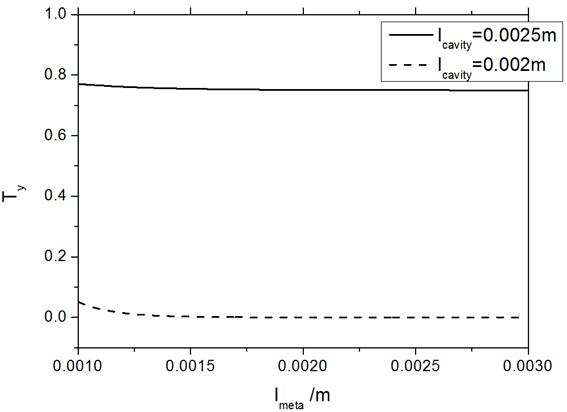Figure 7. The transmissions Ty as a function of the thickness of the anisotropic metamaterial lmeta for a three layer structure for photon in a given frequency f = 40 GHz with lcavity = 0.0025 m (solid line) and lcavity = 0.002 m (dashed line).

4. Conclusion

Based on the input-output relations of the polarized photon in a multilayer system with the anisotropic metamaterials, we calculated the transmission of polarized photon through a multilayer cavity structure with the anisotropic metamaterials. The results show that the resonant peaks of transmission for certain polarized photons occur in correspondence with a negative value of the permittivity. This indicates the feasibility of applications using cavity structures with the anisotropic metamaterials as filters for certain polarized photons. Furthermore, the transmission of polarized photons having a given frequency can achieve resonant peaks by adjusting the thicknesses of the air and the anisotropic metamaterials. There is the resonant peak appearing no matter what the value of the frequency of the input photon. This means that if the frequency of the input photon is kept as a constant, we can design a suitable cavity to make it resonant through adjusting the thickness of the cavity. We can adjust the resonant peak through changing the layer number. Increasing the layer number the more resonant peaks appear. Increasing the cavity thickness the space of the resonant peaks will decrease if the layer number is a constant.

For a three layer cavity, the resonant peak will appear to a certain cavity thickness when we keep the frequency of the input photon as a constant. As the frequency of the input photon becomes bigger the thickness of the cavity corresponding to the resonant peak becomes smaller. At the same time the resonant peak becomes sharp. This means that the thickness of the cavity is restrictive if the frequency of the input photon is high. If the frequency of the photon is fixed, we can adjust the thickness of the cavity to get the resonant transmission for the polarized photon. The transmission is almost invariant with the changing of the thickness of the anisotropic metamaterials. It means the resonant peak is insensitive to the thickness of the anisotropic metamaterials. So the thickness of the cavity which is the air in our designed cavity is important if you want to design a cavity structure with anisotropic metamaterials to obtain the resonant transmission of the polarized photon. These conclusions will give us some instructions to the design of the cavity structure with the anisotropic metamaterials as the filter of the polarized photon.

Acknowledgements

Project supported by the National Natural Science Foundation of China (Grant No. 11104071) and the Fundamental Research Funds for the Central Universities (Grant No. 2015MS01).

References

1. D. R. Smith, W. J. Padilla, D. C. Vier, S. C. Nemat-Nasser, and S. Schultz, Composite Medium with Simultaneously Negative Permeability and Permittivity, Phys. Rev. Lett. 84 4184 (2000)
2. J. B. Pendry, Negative Refraction Makes a Perfect Lens, Phys. Rev. Lett. 85 3966 (2000)
3. L. F. Shen, S. L. He and S. S. Xiao, Stability and quality factor of a one-dimensional subwavelength cavity resonator containing a left-handed material, Phys. Rev. B 69 115111 (2004)
4. J. Li, L. Zhou, C. T. Chan, P. Sheng, Photonic Band Gap from a Stack of Positive and Negative Index Materials, Phys. Rev. Lett. 90 083901 (2003)
5. D. R. Smith and D. Schurig, Electromagnetic Wave Propagation in Media with Indefinite Permittivity and Permeability Tensors, Phys. Rev. Lett. 90 077405 (2003)
6. L. Zhou, C. T. Chan, and P. Sheng, Anisotropy and oblique total transmission at a planar negative-index interface, Phys. Rev. B 68 115424 (2003)
7. S. Sun, X. Huang, and L. Zhou, Two-dimensional complete photonic gaps from layered periodic structures containing anisotropic left-handed metamaterials, Phys. Rev. E 75 066602 (2007)
8. L. Hu and S. T. Chui, Characteristics of electromagnetic wave propagation in uniaxially anisotropic left-handed materials, Phys. Rev. B 66 085108 (2002)
9. J. Hao, Y. Yuan., L. Ran, T. Jiang, J. A. Kong, C. T. Chan and L. Zhou, Manipulating Electromagnetic Wave Polarizations by Anisotropic Metamaterials, Phys. Rev. Lett. 99 063908 (2007)
10. J. Hao and L. Zhou, Electromagnetic wave scatterings by anisotropic metamaterials: Generalized 4×4 transfer-matrix method, Phys. Rev. B 77 094201 (2008)
11. J. Hao, M. Qiu and L. Zhou, Manipulate light polarizations with metamaterials: From microwave to visible, Front. Phys. 5(3), 291 (2010)
12. H. F. Ma, W. X. Tang, Q. Cheng and T. J. Cui, A single metamaterial plate as bandpass filter, transparent wall, and polarization converter controlled by polarizations Appl. Phys. Lett. 105 081908 (2014)
13. Y. Dong, X. Cui, Quantum optical correlation through metamaterials, Front. Phys. 7 (5), 513 (2012)
14. Y. Dong, X. Zhang, Quantum-optical input–output relations and entanglement distillation by anisotropic planar multilayers, J. Opt. 13 035401 (2011)
15. Y. Dong, C. Liu, Electromagnetic field quantization and input-output relation for anisotropic magnetodielectric metamaterial, Chin. Phys. B 24 064206 (2015)
16. Y. Dong, J. You, Propagation of polarized photons through a cavity with an anisotropic metamaterial, Front. Phys. 11, 114206 (2016)

 Contents 1. 2. 3. 4.
Article ToolsAbstractPDF(339K)